### 8.5.1.3 A sampling-based approach

There are numerous alternative ways to construct a cover. To illustrate the ideas, an approach called the sampling-based neighborhood graph is presented here . Suppose that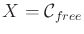, which is a subset of some configuration space. As introduced in Section 5.4, let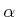be a dense, infinite sequence of samples in. Assume that a collision detection algorithm is available that returns the distance, (5.28), between the robot and obstacles in the world. Such algorithms were described in Section 5.3.

An incremental algorithm is given in Figure 8.17. Initially,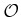is empty. In each iteration, if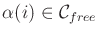and it is not already contained in some neighborhood, then a new neighborhood is added to. The two main concerns are 1) how to define a new neighborhood,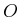, such that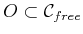, and 2) when to terminate. At any given time, the cover is approximate. The union of all neighborhoods is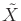, which is a strict subset of. In comparison to Figure 8.15, the cover is a special case in which the neighborhoods do not extend beyond.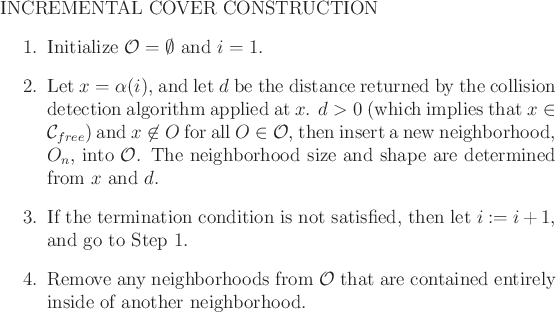Subsections
Steven M LaValle 2020-08-14